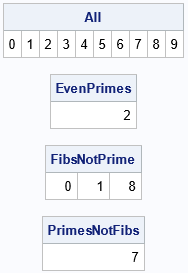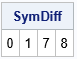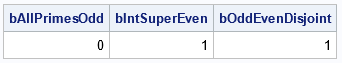The fundamental operations on sets are union, intersection, and set difference, all of which are supported directly in the SAS IML language. While studying another programming language, I noticed that the language supports an additional operation, namely the symmetric difference between two sets. The language also supports query functions to test for subsets, supersets, and disjoint sets. This article shows how to work with sets in the SAS IML language, including how to compute the symmetric difference and how to implement set queries.

### Support for sets in SAS

The SAS IML language has four built-in functions that operate on sets:

1. Union: The UNION function computes the union of sets.
2. Intersection: The XSECT function computes the intersection of sets.
3. Difference: The SETDIF function computes the difference between two sets.
4. Subset: The ELEMENT function returns an indicator variable that specifies which elements of one vector are contained in another.

In a previous article, I showed how to test whether two sets are equal.

To show how the usual set operations work, let's create four sets of integers restricted to the range [0, 9]. The four sets are:

• The even numbers, which form the set {0, 2, 4, 6, 8}.
• The odd numbers, which form the set {1, 3, 5, 7, 9}.
• The prime numbers, which form the set {2, 3, 5, 7}.
• The Fibonacci numbers. The Fibonacci sequence is 0, 1, 1, 2, 3, 5, 8, ..., so the set of Fibonacci numbers less than 10 is the set {0, 1, 2, 3, 5, 8}. You can use the UNIQUE function to create a set of unique, sorted, values from an arbitrary vector of values.
```proc iml; Evens = {0, 2, 4, 6, 8}; Odds = {1, 3, 5, 7, 9}; Primes = {2, 3, 5, 7}; /* Note: if values are not distinct, use UNIQUE function to create a set */ Fibonaccis = unique({0, 1, 1, 2, 3, 5, 8});   /* union */ All = union( Evens, Odds );   /* intersection */ EvenPrimes = xsect( Evens, Primes );   /* set difference A \ B */ FibsNotPrime = setdif( Fibonaccis, Primes ); PrimesNotFibs = setdif( Primes, Fibonaccis ); print All, EvenPrimes, FibsNotPrime, PrimesNotFibs;```The program demonstrates a few simple set operations:

• The UNION function computes the union of the even and odd numbers, which results in all the whole numbers (less than 10).
• The XSECT function computes the intersection of the even numbers and the primes. The number 2 is the only even prime.
• Whereas unions and intersections are commutative operations, the set difference is not. The first call to the SETDIF function computes the set of elements that are Fibonacci numbers but not prime. This is the set {0, 1, 8}. The second call computes the set of primes (less than 10) that are not Fibonacci numbers. This is the set {7}.

### The symmetric difference between sets

The symmetric difference between two sets, A and B, is the set of elements that are in either A or B, but not both. In other words, you take the union of the sets and remove any elements in the intersection: (A ∪ B) \ (A ∩ B). In computer science and logic, this is sometimes called the "exclusive OR" (XOR) operation. Clearly, you can compute this set in SAS IML by using the union, intersection, and set-difference operators, as follows:

```/* symmetric difference is the union minus the intersection: (A U B) \ (A & B) */ start SymDiff(A, B); U = union(A,B); N = xsect(A,B); return setdif(U, N); finish;   SymDiff = SymDiff( Fibonaccis, Primes ); print SymDiff;```The symmetric difference is commutative. The example shows the set of integers that are either Fibonacci numbers or are prime, but not both. The result is the set {0, 1, 7, 8}, which is also the union of the differences A\B and B\A.

### Subsets, supersets, and disjoint sets

So far, we have not used the ELEMENT function. The ELEMENT function indicates which elements in one set are contained in another set. In other words, it identifies the elements of a set A that are also in another set B. You can use the ELEMENT function to determine whether all the elements in A are also elements of B (that is, whether A ⊆ B). By exchanging the roles of A and B, you can determine whether A ⊇ B. Lastly, two sets are disjoint if their intersection is empty. All of these queries can be written in the SAS IML language in a natural way:

```/* isSubset: return 1 (true) if A is a subset of B */ start isSubset(A, B); AinB = element(A,B); return( all(AinB) ); finish;   /* isSubset: return 1 (true) if A is a superset of B */ start isSuperset(A, B); return isSubset(B,A); finish;   /* isDisjoint: return 1 (true) if A does not intersect B */ start isDisjoint(A, B); return( isEmpty(xsect(A,B)) ); finish;   /* run some examples */ bAllPrimesOdd = isSubset( Primes, Odds ); /* are the primes a subset of the odd numbers? (no) */ bIntSuperEven = isSuperset( All, Evens ); /* are the integers a superset of the odds? (yes) */ bOddEvenDisjoint = isDisjoint( Odds, Evens ); /* are the odds and evens disjoint? (yes) */ print bAllPrimesOdd bIntSuperEven bOddEvenDisjoint;```The output shows the following:

• The prime numbers are not a subset of the odd numbers because 2 is a prime but not an odd number.
• The set of all integers is a superset of the set of even numbers.
• The odd numbers and even numbers are disjoint sets.

### Summary

The SAS IML language supports several built-in operations for unions, intersections, differences, and set membership. You can use the built-in operations to create new operations such as the symmetric difference (XOR) or to query the relationship between two sets. By using these functions, you can experience the joy of sets.

The post The joy of sets appeared first on The DO Loop.